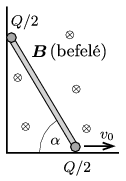Mathematical and Physical Journal
for High Schools
Issued by the MATFUND Foundation
 Already signed up? New to KöMaL?

#Problem P. 4840. (April 2016)

P. 4840. A thin rod of length $\displaystyle L$ is moved in a vertical plane in a right corner such that the speed of the lower, horizontally moving end of the rod has a constant speed of $\displaystyle v_0$, and the other end touches the vertical wall at every moment. At both ends of the rod there are two small charged spheres of charge $\displaystyle Q/2$ (one ball at each end). The whole system is in uniform magnetic field of magnetic induction $\displaystyle \boldsymbol B$. The magnetic induction is horizontal and perpendicular to the plane of the figure pointing into the paper.$\displaystyle a)$ What is the magnitude and the direction of the force exerted on the rod by the magnetic field, when the rod makes an angle of $\displaystyle \alpha$ with the horizontal?

$\displaystyle b)$ Determine this force in the same position of the rod, if there are no charged spheres at the ends of the rod, but there is only one charged sphere at the midpoint of the rod. The charge on this sphere is $\displaystyle Q$.

(5 pont)

Deadline expired on May 10, 2016.

### Statistics:

 34 students sent a solution. 5 points: Asztalos Bogdán, Balogh Menyhért, Bekes Nándor, Büki Máté, Csorba Benjámin, Di Giovanni András, Fehér 169 Szilveszter, Fekete Balázs Attila, Forrai Botond, Ghada Alshalan, Iván Balázs, Jakus Balázs István, Kasza Bence, Kovács Péter Tamás, Körmöczi Dávid, Mány Bence, Marozsák Tóbiás , Németh Flóra Boróka, Olosz Adél, Sal Kristóf, Szántó Benedek, Szentivánszki Soma , Tomcsányi Gergely. 4 points: Blum Balázs, Gémes Antal, Krasznai Anna, Makovsky Mihály, Nagy 555 Botond, Németh 777 Róbert, Páhoki Tamás, Tófalusi Ádám. 3 points: 3 students.

Problems in Physics of KöMaL, April 2016